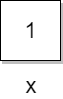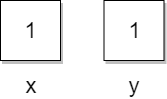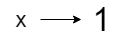# Concept of Variable in Python

Before understanding the concept of variables in Python, you have to know how variables work in other programming languages. In C, Java, and many other programming languages, the concept of a variable is linked to memory space, and a variable is perceived as a storage box that can store some value.

Example:
``x = 1;``

In the above example, memory is allocated with the name x, and there the value 1 is stored. Here, we can see the memory as a container that stores the value, as shown in the picture below.This way, for each variable, there will be a new memory space created with the name of the variable, and if we change the value of the variable, then memory will be updated with the new value.

Example:
``int x,y = 1;``In this way, we can understand how the variable works in other programming languages. But in Python, the case is different, and here a variable is seen as a tag or name that is tied to some value.

Example:
``x = 1``

Here, in the above Python example, a value 1 is created in the memory, and then the tag name x has been created, which is tied to the value.If we change the variable value to a new value, then a new value is created in memory, and the tag is shifted to a new value. In this case, the old value becomes un-referenced, and the garbage collector removes it.

Assigning a variable to another variable creates a new tag connected to the same value.Example:
``int y = x;``

In the above example, we are storing the value of x in y. A new tag y will be generated, which will refer to the value 1.

In this way, we can understand how variables in Python differ from other programming languages.